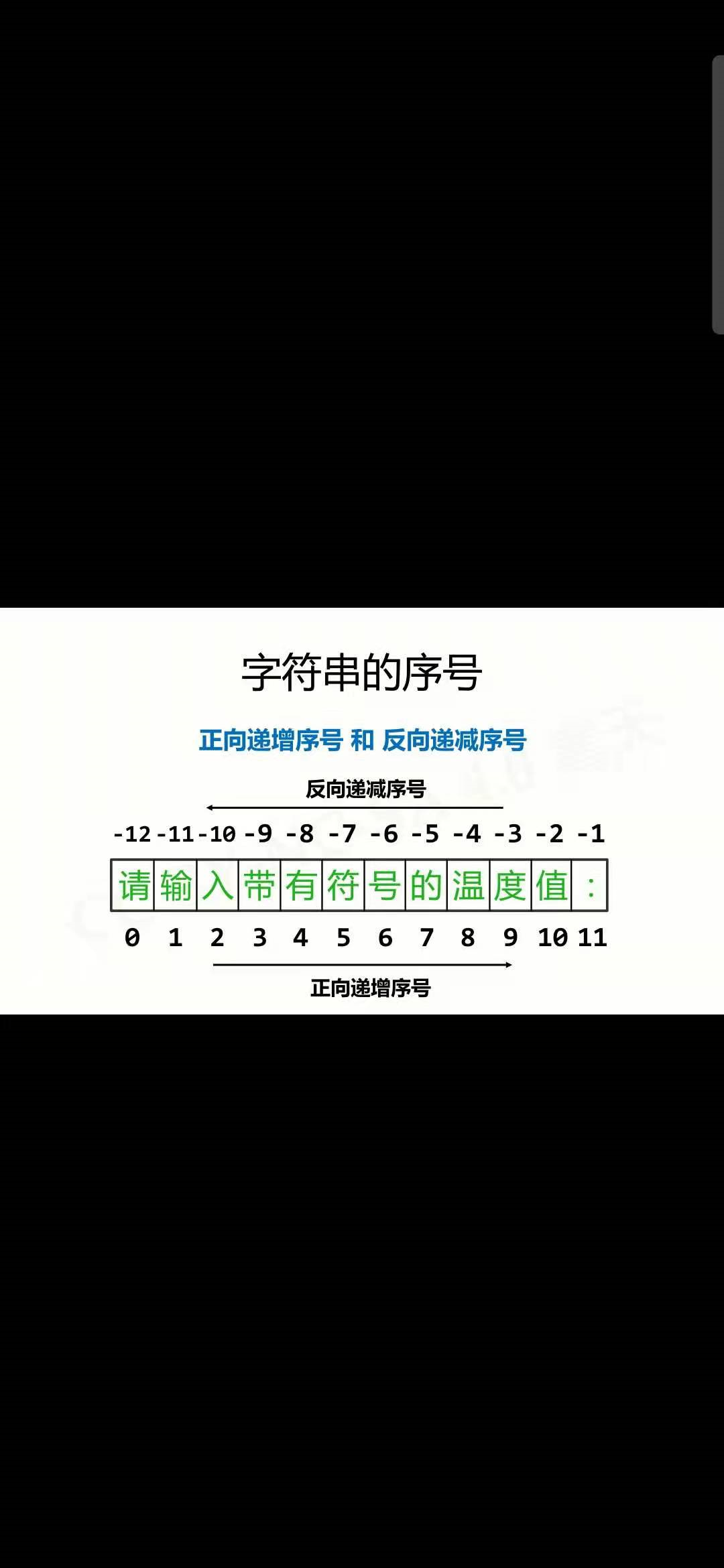# python北理工（第一章）

• 1.1程序设计基础方法
• 1.2python开发环境配置
• 1.3实例一：温度转换
• 1.4python语法元素分析

1.1程序设计的基本方法

Intel公司创始人之一戈登·摩尔在1965年提出

CPU/GPU、内存，硬件，电子产品价格等都遵循摩尔定律

c诞生于1972年，它是第一个被广泛使用的编程语言
python语言诞生于1990年，它是最流行最好用的编程语言

IPO（input- process - output）

python概述
python（蟒蛇），拥有者是python software Foundation(PSF),非盈利组织，致力于保护python语言开放，开源和发展。
2002年python2
2008年python3
python的下载（点击）
（分为32位和64位）

python的两种编程方式

r = 25
area = 3.1415 * r * r
print(area)
print("{:.2f}".format(area))#知识点是print的格式化输出，先观察，以后会进行细致的讲解


import turtle
#用到标准库turtle库
turtle.pensize(2)
turtle.circle(10)
turtle.circle(40)
turtle.circle(80)
turtle.circle(160)



from turtle import *
color('red','red')
begin_fill()
for i in range(5):
fd(200)
rt(144)

end_fill()
done()


C = （F - 32 ）/1.8
F = C * 1.8 + 32

#TempConvert.py
TemStr = input("请输入带有符号的温度值：")
if TemStr[-1] in ['f','F']:
C = (eval(TemStr[0:-1]) - 32)/1.8
print("转换后的温度是{:.2f}".format(C))

elif TemStr[-1] in ['C','c']:
F = 1.8*eval(TemStr[0:-1]) + 32
print("转换后的温度是：{:.2f}".format(F))

else :
print("输入的格式错误")


python程序的语法元素分析

python程序的输入输出
“温度转换”代码分析

python语言由35个保留字

and，elif,import,raise,global,as,else,in,return, nonlocal, assert, except, is, try, True, break, finally, lambda, while, False, class, for, not, with, None, contiue, from, or, yield, async, def, if, pass, del, awaitinput()函数

<变量> = input(<提示信息字符串>)

（这里很重要，你如果没看懂就问我）

print()函数

print()函数的格式化

eval()函数，评估函数

python程序语法元素分析

©️2019 CSDN 皮肤主题: 数字20 设计师: CSDN官方博客